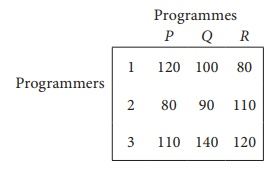Home | | Business Maths 12th Std | Exercise 10.2: Assignment problems(Hungarian Method)

# Exercise 10.2: Assignment problems(Hungarian Method)

Book back answers and solution for Exercise questions - Maths: Operations Research: Assignment problems(Hungarian Method)

Exercise 10.2

1. What is the Assignment problem?

2. Give mathematical form of assignment problem.

3. What is the difference between Assignment Problem and Transportation Problem?

4. Three jobs A, B and C one to be assigned to three machines U, V and W. The processing cost for each job machine combination is shown in the matrix given below. Determine the allocation that minimizes the overall processing cost.5. A computer centre has got three expert programmers. The centre needs three application programmes to be developed. The head of the computer centre, after studying carefully the programmes to be developed, estimates the computer time in minitues required by the experts to the application programme as follows.Assign the programmers to the programme in such a way that the total computer time is least.

6. A departmental head has four subordinates and four tasks to be performed. The subordinates differ in efficiency and the tasks differ in their intrinsic difficulty. His estimates of the time each man would take to perform each task is given belowHow should the tasks be allocated to subordinates so as to minimize the total man-hours?

7. Find the optimal solution for the assignment problem with the following cost matrix.8. Assign four trucks 1, 2, 3 and 4 to vacant spaces A, B, C, D, E and F so that distance travelled is minimized. The matrix below shows the distance.4. 46

5. 280

6. 41 Hours

7. 37

8. 12

Tags : Problem Questions with Answer, Solution , 12th Business Maths and Statistics : Chapter 10 : Operations Research
Study Material, Lecturing Notes, Assignment, Reference, Wiki description explanation, brief detail
12th Business Maths and Statistics : Chapter 10 : Operations Research : Exercise 10.2: Assignment problems(Hungarian Method) | Problem Questions with Answer, Solution